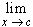#Interactive Real Analysis

Next | Previous | Glossary | Map

## 6.5. Differentiable Functions

### Examples 6.5.6(a):

The function f(x) = | x | is continuous everywhere. Is it also differentiable everywhere ?

We know that f is continuous. To check for differentiability, we have to employ the basic definition:

• f'(x) ==( | x | - | c | ) / (x - c)
• If c > 0 then x > 0 eventually. Then there is no need for the absolute value. The limit become +1.
• If c < 0 then x < 0 eventually. The absolute values are resolved by an additional negative sign. The limit becomes -1.
• If c = 0, then the left and the right-handed limits will be different (-1 and +1). Therefore, the function is not differentiable at 0.

 Your browser can not handle Java applets This is an example of a function that shows that differentiability is a stronger concept than continuity: every differentiable function is continuous (theorem) there are continuous functions that are not differentiable
Next | Previous | Glossary | Map